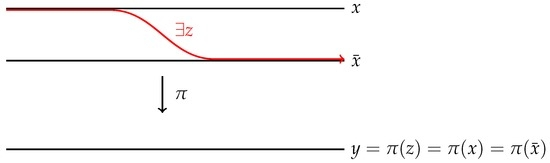Next Article in Journal
CoFea: A Novel Approach to Spam Review Identification Based on Entropy and Co-Training
Next Article in Special Issue
Topological Entropy Dimension and Directional Entropy Dimension for ℤ2-Subshifts
Previous Article in Journal
On Macrostates in Complex Multi-Scale Systems
Previous Article in Special Issue
Positive Sofic Entropy Implies Finite Stabilizer
Open AccessArticle

# Fiber-Mixing Codes between Shifts of Finite Type and Factors of Gibbs Measures

byUijin JungDepartment of Mathematics, Ajou University, 206 Worldcup-ro, Suwon 16499, Korea
Entropy 2016, 18(12), 428; https://doi.org/10.3390/e18120428
Received: 7 October 2016 / Revised: 20 November 2016 / Accepted: 24 November 2016 / Published: 30 November 2016
(This article belongs to the Special Issue Entropic Properties of Dynamical Systems)
A sliding block code $π : X → Y$ between shift spaces is called fiber-mixing if, for every x and $x ′$ in X with $y = π ( x ) = π ( x ′ )$ , there is $z ∈ π - 1 ( y )$ which is left asymptotic to x and right asymptotic to $x ′$ . A fiber-mixing factor code from a shift of finite type is a code of class degree 1 for which each point of Y has exactly one transition class. Given an infinite-to-one factor code between mixing shifts of finite type (of unequal entropies), we show that there is also a fiber-mixing factor code between them. This result may be regarded as an infinite-to-one (unequal entropies) analogue of Ashley’s Replacement Theorem, which states that the existence of an equal entropy factor code between mixing shifts of finite type guarantees the existence of a degree 1 factor code between them. Properties of fiber-mixing codes and applications to factors of Gibbs measures are presented. View Full-Text
Show FiguresFigure 1

MDPI and ACS Style

Jung, U. Fiber-Mixing Codes between Shifts of Finite Type and Factors of Gibbs Measures. Entropy 2016, 18, 428.